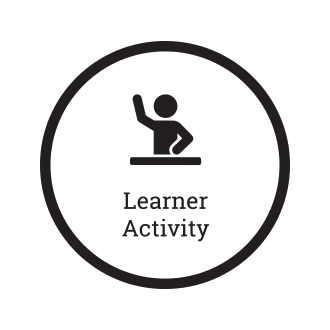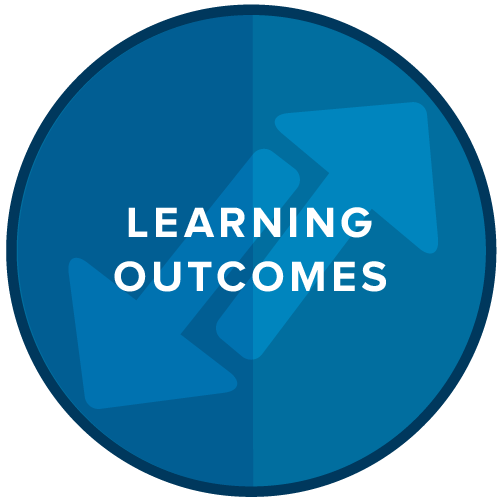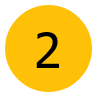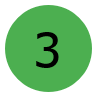Computing## Lesson Video 1

•Watch the Lesson video
Make notes on your notes sheet.
•Make notes on your notes sheet.
•Complete the learning activities
Make any notes on your notes sheet.
•Check the Learning outcomes

## What do I need to Learn?

 I need to learn how to understand and explain the term algorithm.

## Key Terms

Algorithm Sequence Algorithmic thinking

## An Algorithm is Like a Recipe

An algorithm is simply a sequence of steps used to solve a problem, like a recipe. See right. Our recipe might tell us how to create an apple pie or lemon cake.

To get a computer to do something we have to tell it what to do. We do this by using a set of instructions. These instructions are called algorithms.

An algorithm has a defined outcome – a defined end. Algorithms can be written in many different ways. Each way has a different cost, different speed and requires different external hardware.

### A Recipe## Algorithms in programming

You are creating an algorithm every time you write a program. You are creating a set of steps to perform a task. Before we create a computer program we write it out in one of two ways.

1. As a set of intructions like a recipe.

2. As a flowchart.

### Different wasy to create an algorithm## Creating an algorithm### Task 1: Find the error.

#### The following pseudocode algorithm has an error. The program is suppose to ask the user for the length and width of a rectangular room, and then display the room's area. the program must multiply the width by the length in order to deteremine the area.

```area= width x length.
display "what is the room's width?".
input width.
display "what is the room's length?".
input length.
display area.
```

### Task 2: Create an algorithm## Learning Outcomes

•I can explain what an algorithm is.
•I can read an algorithm and find any errors in it.
•I can create my own algorithm to solve a problem.